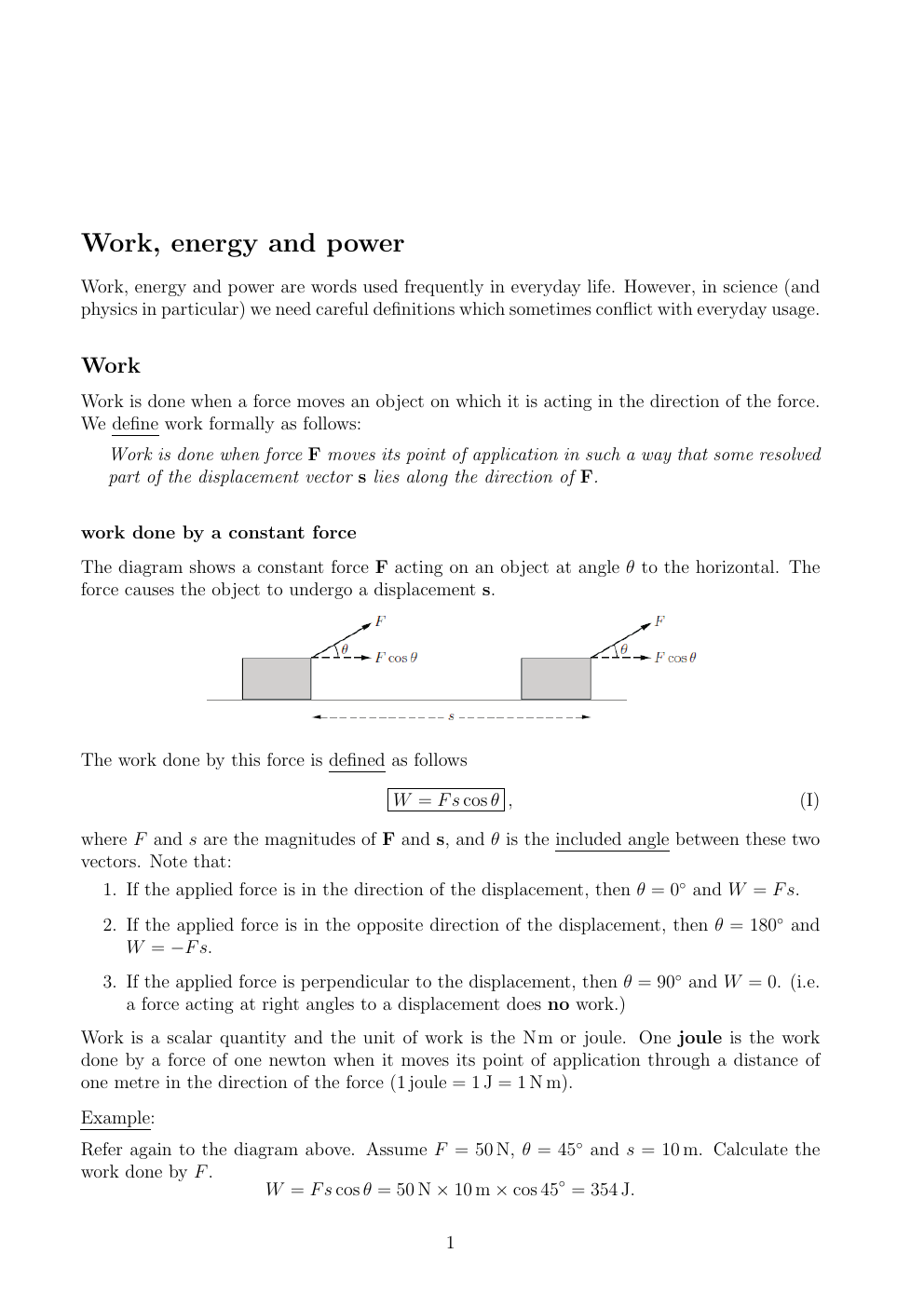# Work Energy & Power

Publié le 21/06/2022

## Extrait du document

« Work, energy and power Work, energy and power are words used frequently in everyday life.

However, in science (and physics in particular) we need careful definitions which sometimes conflict with everyday usage. Work Work is done when a force moves an object on which it is acting in the direction of the force. We define work formally as follows: Work is done when force F moves its point of application in such a way that some resolved part of the displacement vector s lies along the direction of F. work done by a constant force The diagram shows a constant force F acting on an object at angle θ to the horizontal.

The force causes the object to undergo a displacement s. The work done by this force is defined as follows W = F s cos θ , (I) where F and s are the magnitudes of F and s, and θ is the included angle between these two vectors.

Note that: 1.

If the applied force is in the direction of the displacement, then θ = 0◦ and W = F s. 2.

If the applied force is in the opposite direction of the displacement, then θ = 180◦ and W = −F s. 3.

If the applied force is perpendicular to the displacement, then θ = 90◦ and W = 0.

(i.e. a force acting at right angles to a displacement does no work.) Work is a scalar quantity and the unit of work is the Nm or joule.

One joule is the work done by a force of one newton when it moves its point of application through a distance of one metre in the direction of the force (1 joule = 1 J = 1 N m). Example: Refer again to the diagram above.

Assume F = 50 N, θ = 45◦ and s = 10 m.

Calculate the work done by F . W = F s cos θ = 50 N × 10 m × cos 45◦ = 354 J. 1. »

↓↓↓ APERÇU DU DOCUMENT ↓↓↓